1. /
2. CBSE
3. /
4. Class 09
5. /
6. Mathematics
7. /
8. NCERT Solutions for Class...

# NCERT Solutions for Class 9 Maths Exercise 1.1

NCERT solutions for Class 9 Maths Number Systems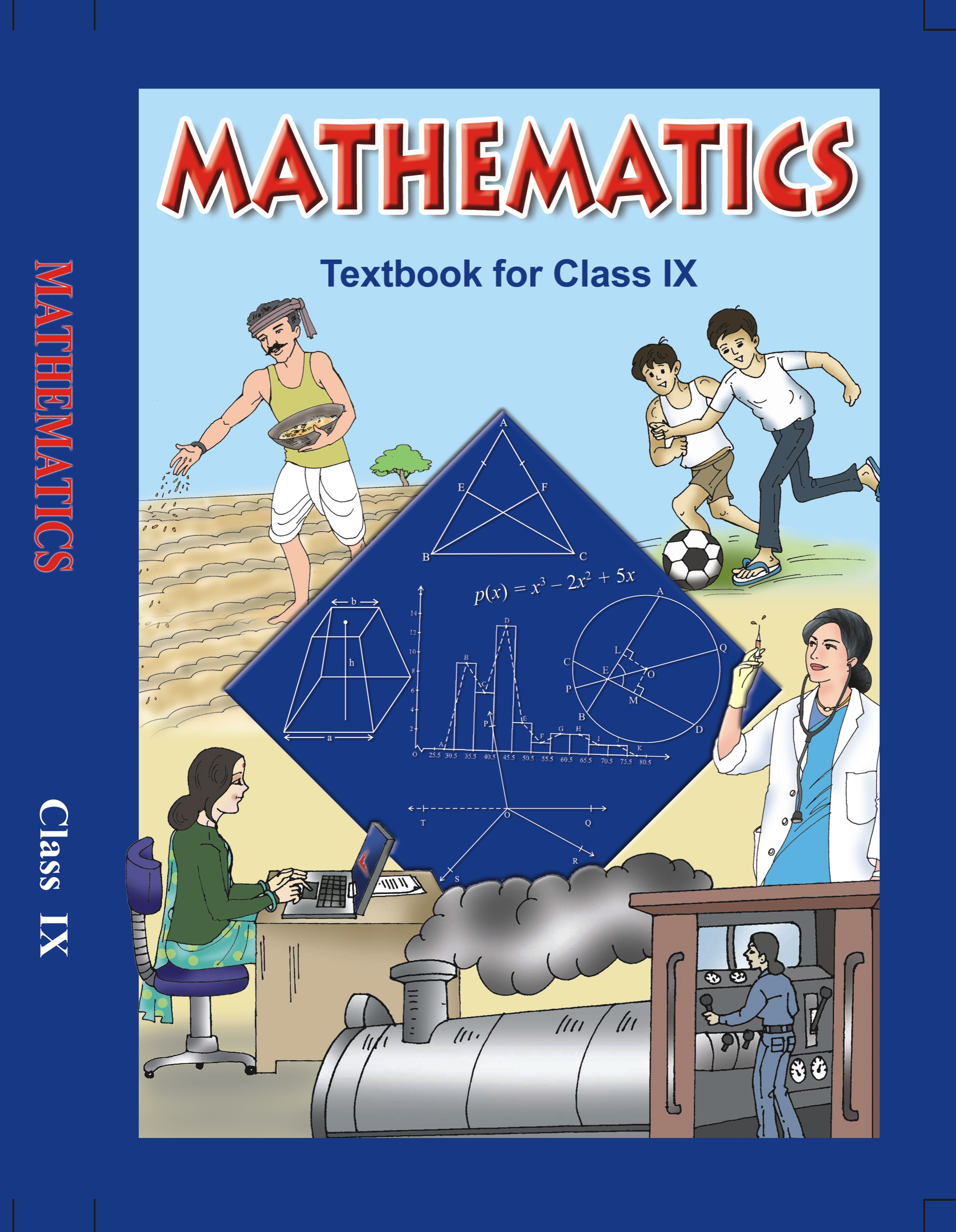## NCERT Solutions for Class 9 Maths Number Systems

###### 1. Is zero a rational number? Can you write it in the form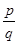, where p and q are integers and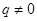?

Ans. Consider the definition of a rational number.

A rational number is the one that can be written in the form of, where p and q are integers and.

Zero can be written as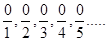.

So, we arrive at the conclusion that 0 can be written in the form of, where q is any integer.

Therefore, zero is a rational number.

NCERT Solutions for Class 9 Maths Exercise 1.1

###### 2. Find six rational numbers between 3 and 4.

Ans. We know that there are infinite rational numbers between any two numbers.

A rational number is the one that can be written in the form of, where p and q are

Integers and.

We know that the numbers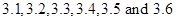all lie between 3 and 4.

We need to rewrite the numbersinform to get the rational numbers between 3 and 4.

So, after converting, we get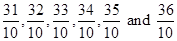.

We can further convert the rational numbers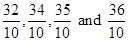into lowest fractions.

On converting the fractions into lowest fractions, we get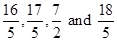.

Therefore, six rational numbers between 3 and 4 are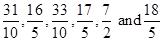.

NCERT Solutions for Class 9 Maths Exercise 1.1

###### 3. Find five rational numbers between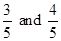.

Ans. We know that there are infinite rational numbers between any two numbers.

A rational number is the one that can be written in the form of, where p and q are

Integers and.

We know that the numberscan also be written as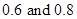.

We can conclude that the numbers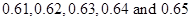all lie betweenWe need to rewrite the numbersinform to get the rational numbers between 3 and 4.

So, after converting, we get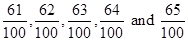.

We can further convert the rational numbers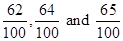into lowest fractions.

On converting the fractions, we get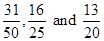.

Therefore, six rational numbers between 3 and 4 are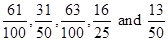.

NCERT Solutions for Class 9 Maths Exercise 1.1

###### 4. State whether the following statements are true or false. Give reasons for your answers.

(i) Every natural number is a whole number.

(ii) Every integer is a whole number.

(iii) Every rational number is a whole number.

Ans. (i) Consider the whole numbers and natural numbers separately.

We know that whole number series is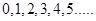.

We know that natural number series is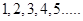.

So, we can conclude that every number of the natural number series lie in the whole number series.

Therefore, we conclude that, yes every natural number is a whole number.

###### (ii) Consider the integers and whole numbers separately.

We know that integers are those numbers that can be written in the form of, where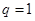.

Now, considering the series of integers, we have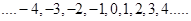.

We know that whole number series is.

We can conclude that all the numbers of whole number series lie in the series of integers. But every number of series of integers does not appear in the whole number series.

Therefore, we conclude that every integer is not a whole number.

###### (iii) Consider the rational numbers and whole numbers separately.

We know that rational numbers are the numbers that can be written in the form, where.

We know that whole number series is.

We know that every number of whole number series can be written in the form ofas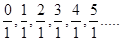.

We conclude that every number of the whole number series is a rational number. But, every rational number does not appear in the whole number series.

Therefore, we conclude that every rational number is not a whole number.

## NCERT Solutions for Class 9 Maths Exercise 1.1

NCERT Solutions Class 9 Maths PDF (Download) Free from myCBSEguide app and myCBSEguide website. Ncert solution class 9 Maths includes text book solutions from Mathematics Book. NCERT Solutions for CBSE Class 9 Maths have total 15 chapters. 9 Maths NCERT Solutions in PDF for free Download on our website. Ncert Maths class 9 solutions PDF and Maths ncert class 9 PDF solutions with latest modifications and as per the latest CBSE syllabus are only available in myCBSEguide.

## CBSE app for Class 9

To download NCERT Solutions for Class 9 Maths, Computer Science, Home Science,Hindi ,English, Social Science do check myCBSEguide app or website. myCBSEguide provides sample papers with solution, test papers for chapter-wise practice, NCERT solutions, NCERT Exemplar solutions, quick revision notes for ready reference, CBSE guess papers and CBSE important question papers. Sample Paper all are made available through the best app for CBSE students and myCBSEguide website.Test Generator

Create question paper PDF and online tests with your own name & logo in minutes.myCBSEguide

Question Bank, Mock Tests, Exam Papers, NCERT Solutions, Sample Papers, Notes